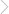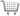## A2 Chemistry: Inorganic & Physical Chemistry (I): General Concepts

### By Mark Wardrop | Copyright Year:2010 | ISBN-13: 9780340974674

Published:27/11/2009These teacher resource packs contain new material that gives teachers alternative activities to help in their teaching of AS chemistry. The pack has been developed for the new specifications for teaching from autumn 2008 and the suggested teaching order is tailored to the new specifications.
• Dramatically cut your preparation time
• Provide teaching schemes which have been proven to work
• Offer complete flexibility, as teaching plans are self-contained
• Provide a solution to staff absence

### CONTENTS

Introduction
Equilibria
Teaching Plan 1 Equilibria
Helpsheet 1.1 The equilibrium law
Helpsheet 1.2 Writing Kc expressions
Helpsheet 1.3 Calculating Kc values
Helpsheet 1.4 Calculating Kc values from experiments
Helpsheet 1.5 Calculating concentrations at equilibrium
Helpsheet 1.6 Effect of changing concentrations on Kc
Helpsheet 1.7 Entropy and equilibrium
Helpsheet 1.8 Partial pressure and Kp
Helpsheet 1.9 Calculations with Kp
Worksheet 1.1 Writing Kc expressions
Worksheet 1.2 Calculating Kc values
Worksheet 1.3 Calculating equilibrium concentrations
Worksheet 1.4 Kc values and position of equilibrium
Worksheet 1.5 Kp calculations
Student Activity 1.1 Determining an equilibrium constant
Practical Notes 1.1 Determining an equilibrium constant
End-of-Topic Test 1 Questions and mark scheme
Kinetics
Teaching Plan 2 Kinetics
Helpsheet 2.1 Rate of reaction
Helpsheet 2.2 Measuring rates of reaction
Helpsheet 2.3 The rate equation and order of reaction
Helpsheet 2.4 Finding orders from graphs
Helpsheet 2.5 Finding orders from initial reaction rates
Helpsheet 2.6 Initial rates versus continuous monitoring
Helpsheet 2.7 Rate-determining step and mechanisms
Helpsheet 2.8 Homogeneous and heterogeneous catalysis
Helpsheet 2.9 Temperature and the rate constant
Helpsheet 2.10 Finding the activation energy
Worksheet 2.1 Measuring rate of reaction
Worksheet 2.2 The rate equation
Worksheet 2.3 Finding order of reaction from graphs
Worksheet 2.4 Finding order of reaction from initial rates
Worksheet 2.5 Rate-determining step and mechanisms
Worksheet 2.6 Temperature, catalysts and the rate constant
Worksheet 2.7 Finding the activation energy
Student Activity 2.1 The reaction between iodine and propanone
Practical Notes 2.1 The reaction between iodine and propanone
Student Activity 2.2 Investigating a catalyst
Practical Notes 2.2 Investigating a catalyst
Student Activity 2.3 Temperature, reaction rate and activation energy
Practical Notes 2.3 Temperature, reaction rate and activation energy
End-of-Topic Test 2 Questions
Acid–base equilibria
Teaching Plan 3 Acid–base equilibria
Helpsheet 3.1 What are acids?
Helpsheet 3.2 Summary of reactions of acids
Helpsheet 3.3 Ionic equations for acid reactions
Helpsheet 3.4 Strong and weak acids or bases
Helpsheet 3.5 Calculating pH of strong acids
Helpsheet 3.6 Ka and calculating pH of weak acids
Helpsheet 3.7 Kw and calculating pH of strong bases
Worksheet 3.1 Acid–base conjugate pairs
Worksheet 3.2 Writing equations for acid reactions
Worksheet 3.3 Strong and weak acids or bases
Worksheet 3.4 Calculating pH of strong acids
Worksheet 3.5 Ka and the pH of weak acids
Worksheet 3.6 Kw and the pH of strong bases
Student Activity 3.1 What are acids?
Student Activity 3.2 pH values of strong and weak acids
Student Activity 3.3 Finding Ka for a weak acid: method 1
End-of-Topic Test 3 Questions
Titrations and buffer solutions
Teaching Plan 4 Titrations and buffer solutions
Helpsheet 4.1 What are buffer solutions?
Helpsheet 4.2 Calculations involving buffer solutions
Helpsheet 4.3 Titration curves
Helpsheet 4.4 Acid–base indicators and titrations
Helpsheet 4.5 Using titration curves to find Ka values
Helpsheet 4.6 Enthalpy change of neutralisation
Worksheet 4.1 Calculations involving buffer solutions
Worksheet 4.2 Titration curves
Worksheet 4.3 Acid–base indicators and titrations
Worksheet 4.4 Titration curves and buffer solutions
Worksheet 4.5 Calculating standard enthalpy change of neutralisation
Student Activity 4.1 What are buffer solutions?
Student Activity 4.2 Making a buffer solution
Student Activity 4.3 Titration curves
Student Activity 4.4 Find Ka for a weak acid: method 2